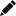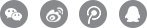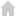当前位置: 心理咨询 > 青少年心理咨询>戒网瘾> 正文

# 网络成瘾自测问卷

0~5的具体含义是：1 = 罕见,2 = 偶尔,3 = 较常,4 = 经常,5 = 总是, 0= 没有

1．你发现你在网上逗留的时间比你原来打算的时间要长?
1=罕见   2=偶尔   3=较常   4=经常   5=总是   0=没有
2. 由于上网上的时间太多,以至于忘记了要做的家务?
1=罕见   2=偶尔   3=较常   4=经常   5=总是   0=没有
3. 你觉得因特网带给你的愉悦超过了亲朋密友之间的亲昵?
1=罕见   2=偶尔   3=较常   4=经常   5=总是   0=没有
4. 你会与网上的人建立各种新的关系?
1=罕见   2=偶尔   3=较常   4=经常   5=总是   0=没有
5. 你的亲友会抱怨你花太长的时间在网上?
1=罕见   2=偶尔   3=较常   4=经常   5=总是   0=没有
6. 由于你花太多的时间在网上,以至于会耽误学业和工作?
1=罕见   2=偶尔   3=较常   4=经常   5=总是   0=没有
7. 你宁愿去查收电子邮件,也不愿去完成必须做的工作?
1=罕见   2=偶尔   3=较常   4=经常   5=总是   0=没有
8. 由于上网,影响了你的学习或工作业绩和效果?
1=罕见   2=偶尔   3=较常   4=经常   5=总是   0=没有
9.你尽量隐瞒你在网上的所作所为?
1=罕见   2=偶尔   3=较常   4=经常   5=总是   0=没有
10. 你会同时想起网上的快乐和生活的烦恼?
1=罕见   2=偶尔   3=较常   4=经常   5=总是   0=没有
11. 在你准备开始上网时,你会觉得自己早就渴望上网了?
1=罕见   2=偶尔   3=较常   4=经常   5=总是   0=没有
12. 没了互联网,生活会变得枯燥,空虚和无聊?
1=罕见   2=偶尔   3=较常   4=经常   5=总是   0=没有
13. 当有人打扰你上网时,你会恼怒或吵闹?
1=罕见   2=偶尔   3=较常   4=经常   5=总是   0=没有
14. 你会为深夜上网而不睡觉?
1=罕见   2=偶尔   3=较常   4=经常   5=总是   0=没有
15. 其它时间你仍全身心想着上网或幻想着上网?
1=罕见   2=偶尔   3=较常   4=经常   5=总是   0=没有
16. 你上网时老想着"就再多上一会儿"?
1=罕见   2=偶尔   3=较常   4=经常   5=总是   0=没有
17. 你尝试减少上网时间但却失败了?
1=罕见   2=偶尔   3=较常   4=经常   5=总是   0=没有
18. 你企图掩饰自己上网的时间?
1=罕见   2=偶尔   3=较常   4=经常   5=总是   0=没有
19. 你选择花更多时间上网,而不是和别人出去玩?
1=罕见   2=偶尔   3=较常   4=经常   5=总是   0=没有
20.当外出不能上网时,你会感到沮丧、忧郁或焦虑,但一上了网,这些感觉就消失了?
1=罕见   2=偶尔   3=较常   4=经常   5=总是   0=没有

20 ~49 分: 你是个一般上网者,只是有时会上的多一些,但总体上是能够自我控制的,尚未沉溺于此。
50 ~79 分：你由于上网似乎开始引起了一些问题,应该谨慎对待上网给你带来的影响,以及对家庭和其他成员带来的影响。
80 ~100 分: 上网已经给你和你的家庭带来了很多问题,你必须马上正视并解决这些问题。

## 戒网瘾## 青少年心理咨询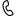咨询电话
0571-86433196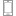手机咨询
13306538268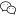微信咨询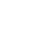留言咨询0571-86433196